# Investment_chapter-1-2020.pdf

30. May 2023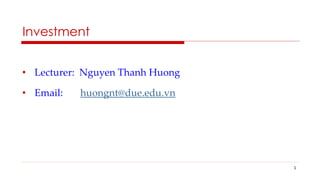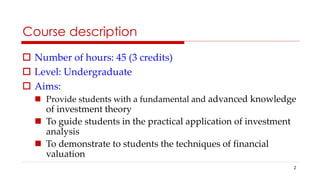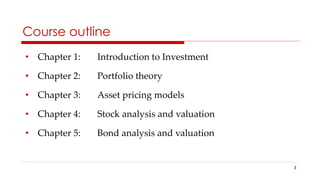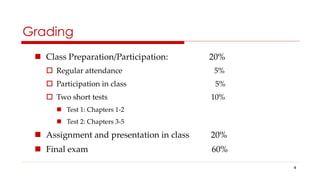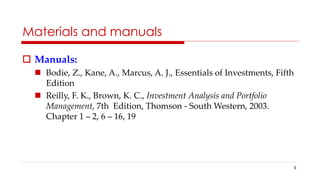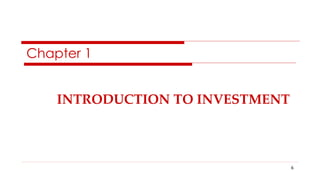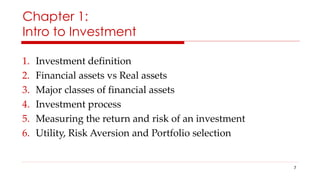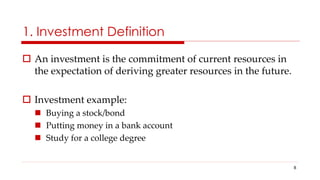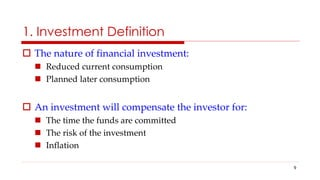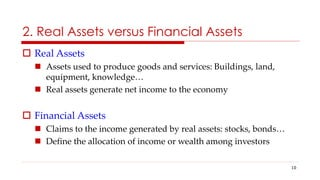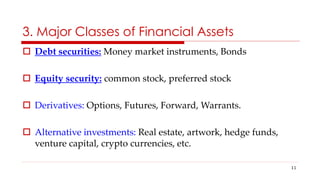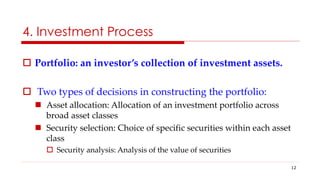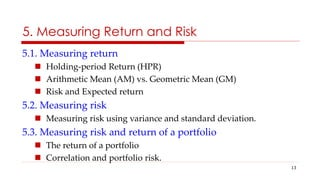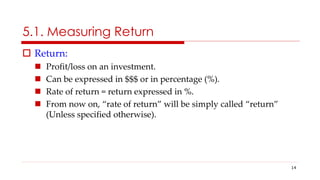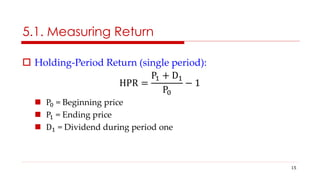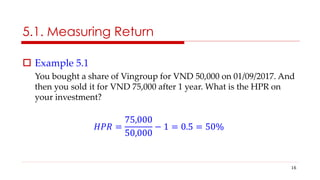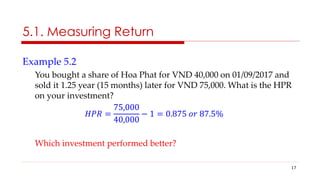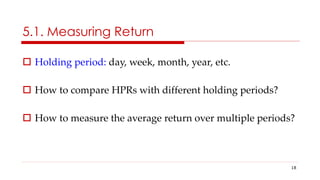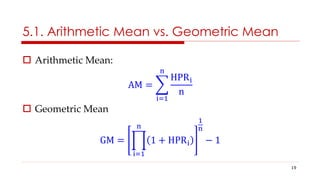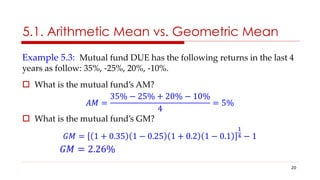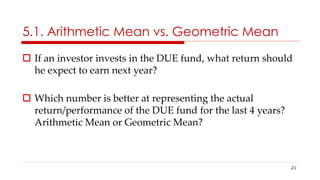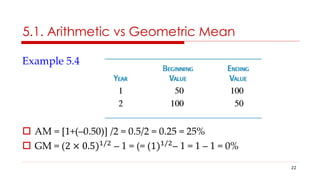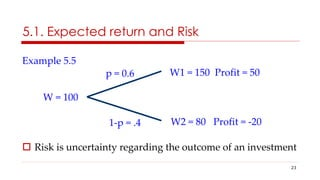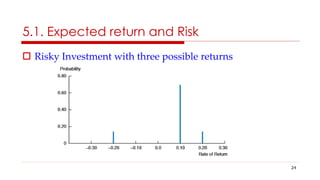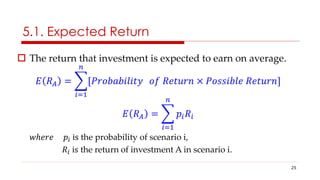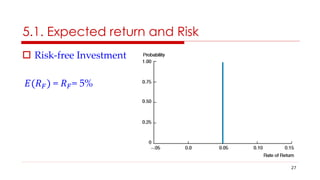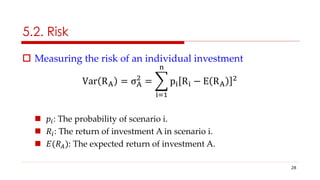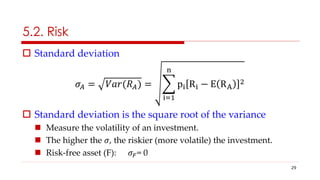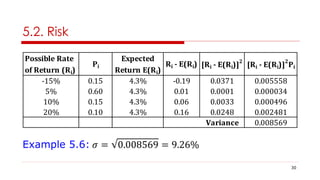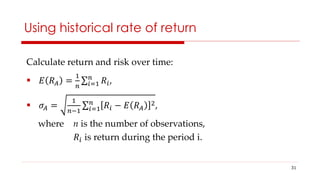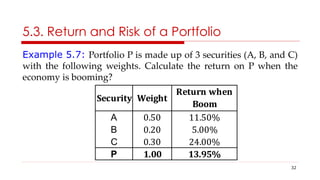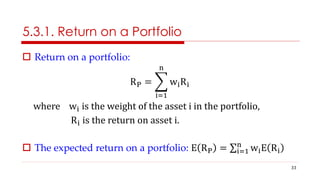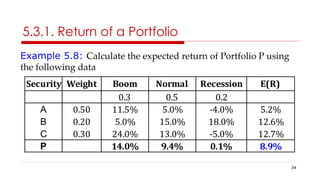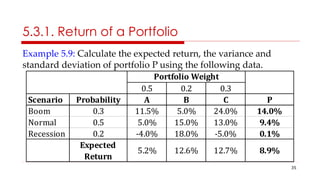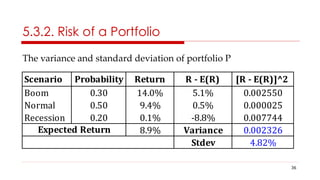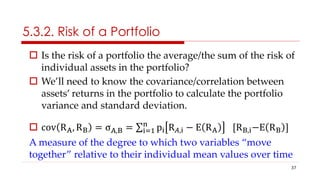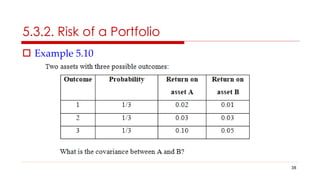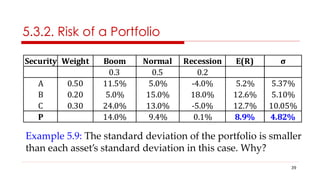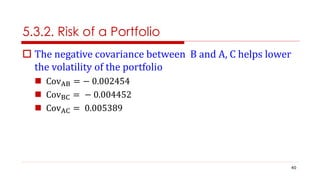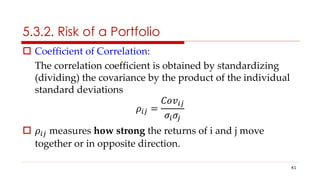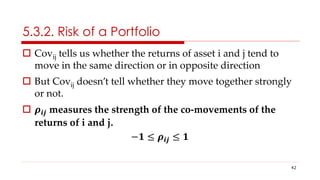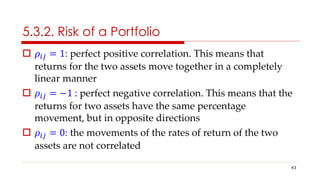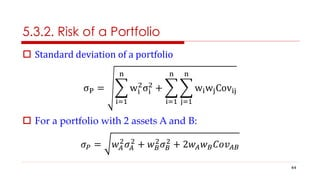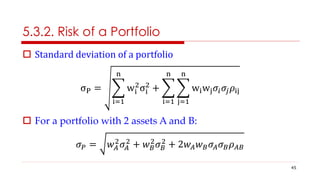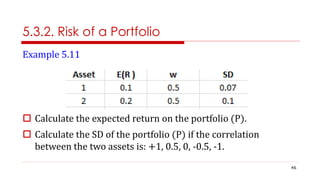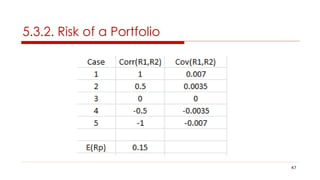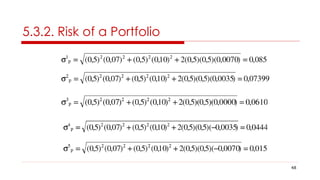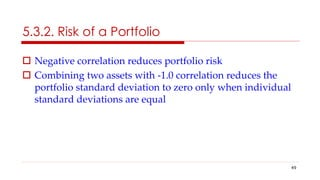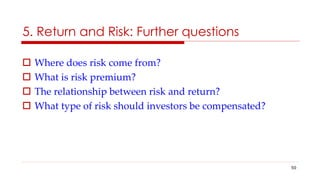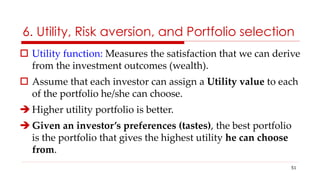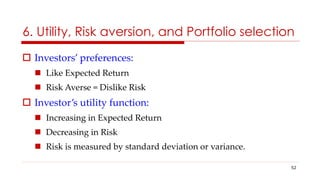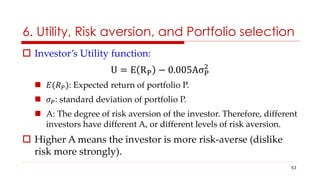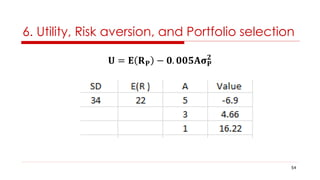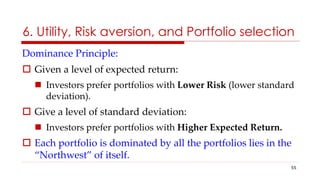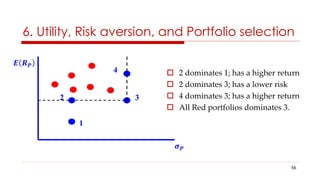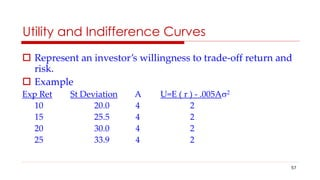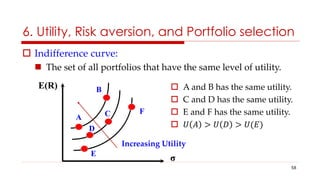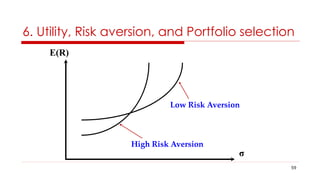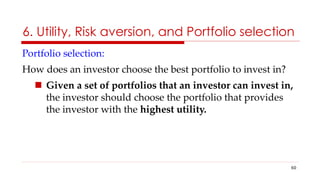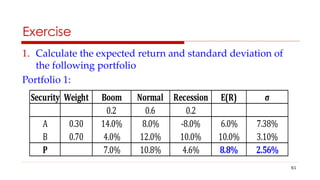1 von 61

### Investment_chapter-1-2020.pdf

• 1. Investment • Lecturer: Nguyen Thanh Huong • Email: huongnt@due.edu.vn 1
• 2. Course description  Number of hours: 45 (3 credits)  Level: Undergraduate  Aims:  Provide students with a fundamental and advanced knowledge of investment theory  To guide students in the practical application of investment analysis  To demonstrate to students the techniques of financial valuation 2
• 3. Course outline • Chapter 1: Introduction to Investment • Chapter 2: Portfolio theory • Chapter 3: Asset pricing models • Chapter 4: Stock analysis and valuation • Chapter 5: Bond analysis and valuation 3
• 4. Grading  Class Preparation/Participation: 20%  Regular attendance 5%  Participation in class 5%  Two short tests 10%  Test 1: Chapters 1-2  Test 2: Chapters 3-5  Assignment and presentation in class 20%  Final exam 60% 4
• 5. 5 Materials and manuals  Manuals:  Bodie, Z., Kane, A., Marcus, A. J., Essentials of Investments, Fifth Edition  Reilly, F. K., Brown, K. C., Investment Analysis and Portfolio Management, 7th Edition, Thomson - South Western, 2003. Chapter 1 – 2, 6 – 16, 19
• 6. INTRODUCTION TO INVESTMENT Chapter 1 6
• 7. Chapter 1: Intro to Investment 1. Investment definition 2. Financial assets vs Real assets 3. Major classes of financial assets 4. Investment process 5. Measuring the return and risk of an investment 6. Utility, Risk Aversion and Portfolio selection 7
• 8. 1. Investment Definition  An investment is the commitment of current resources in the expectation of deriving greater resources in the future.  Investment example:  Buying a stock/bond  Putting money in a bank account  Study for a college degree 8
• 9. 1. Investment Definition  The nature of financial investment:  Reduced current consumption  Planned later consumption  An investment will compensate the investor for:  The time the funds are committed  The risk of the investment  Inflation 9
• 10. 2. Real Assets versus Financial Assets  Real Assets  Assets used to produce goods and services: Buildings, land, equipment, knowledge…  Real assets generate net income to the economy  Financial Assets  Claims to the income generated by real assets: stocks, bonds…  Define the allocation of income or wealth among investors 10
• 11.  Debt securities: Money market instruments, Bonds  Equity security: common stock, preferred stock  Derivatives: Options, Futures, Forward, Warrants.  Alternative investments: Real estate, artwork, hedge funds, venture capital, crypto currencies, etc. 3. Major Classes of Financial Assets 11
• 12. 4. Investment Process  Portfolio: an investor’s collection of investment assets.  Two types of decisions in constructing the portfolio:  Asset allocation: Allocation of an investment portfolio across broad asset classes  Security selection: Choice of specific securities within each asset class  Security analysis: Analysis of the value of securities 12
• 13. 5. Measuring Return and Risk 5.1. Measuring return  Holding-period Return (HPR)  Arithmetic Mean (AM) vs. Geometric Mean (GM)  Risk and Expected return 5.2. Measuring risk  Measuring risk using variance and standard deviation. 5.3. Measuring risk and return of a portfolio  The return of a portfolio  Correlation and portfolio risk. 13
• 14. 5.1. Measuring Return  Return:  Profit/loss on an investment.  Can be expressed in \$\$\$ or in percentage (%).  Rate of return = return expressed in %.  From now on, “rate of return” will be simply called “return” (Unless specified otherwise). 14
• 15. 5.1. Measuring Return  Holding-Period Return (single period): HPR = P1 + D1 P0 − 1  P0 = Beginning price  P1 = Ending price  D1 = Dividend during period one 15
• 16. 5.1. Measuring Return  Example 5.1 You bought a share of Vingroup for VND 50,000 on 01/09/2017. And then you sold it for VND 75,000 after 1 year. What is the HPR on your investment? 𝐻𝑃𝑅 = 75,000 50,000 − 1 = 0.5 = 50% 16
• 17. 5.1. Measuring Return Example 5.2 You bought a share of Hoa Phat for VND 40,000 on 01/09/2017 and sold it 1.25 year (15 months) later for VND 75,000. What is the HPR on your investment? 𝐻𝑃𝑅 = 75,000 40,000 − 1 = 0.875 𝑜𝑟 87.5% Which investment performed better? 17
• 18. 5.1. Measuring Return  Holding period: day, week, month, year, etc.  How to compare HPRs with different holding periods?  How to measure the average return over multiple periods? 18
• 19. 5.1. Arithmetic Mean vs. Geometric Mean  Arithmetic Mean: AM = HPRi n n i=1  Geometric Mean GM = 1 + HPRi n i=1 1 n − 1 19
• 20. 5.1. Arithmetic Mean vs. Geometric Mean Example 5.3: Mutual fund DUE has the following returns in the last 4 years as follow: 35%, -25%, 20%, -10%.  What is the mutual fund’s AM? 𝐴𝑀 = 35% − 25% + 20% − 10% 4 = 5%  What is the mutual fund’s GM? 𝐺𝑀 = 1 + 0.35 1 − 0.25 1 + 0.2 1 − 0.1 1 4 − 1 𝐺𝑀 = 2.26% 20
• 21. 5.1. Arithmetic Mean vs. Geometric Mean  If an investor invests in the DUE fund, what return should he expect to earn next year?  Which number is better at representing the actual return/performance of the DUE fund for the last 4 years? Arithmetic Mean or Geometric Mean? 21
• 22. 5.1. Arithmetic vs Geometric Mean Example 5.4  AM = [1+(–0.50)] /2 = 0.5/2 = 0.25 = 25%  GM = (2 × 0.5)1/2 – 1 = (= (1)1/2 – 1 = 1 – 1 = 0% 22
• 23. 5.1. Expected return and Risk Example 5.5  Risk is uncertainty regarding the outcome of an investment W = 100 W1 = 150 Profit = 50 W2 = 80 Profit = -20 1-p = .4 23
• 24.  Risky Investment with three possible returns 5.1. Expected return and Risk 24
• 25.  The return that investment is expected to earn on average. 𝐸 𝑅𝐴 = [𝑃𝑟𝑜𝑏𝑎𝑏𝑖𝑙𝑖𝑡𝑦 𝑛 𝑖=1 𝑜𝑓 𝑅𝑒𝑡𝑢𝑟𝑛 × 𝑃𝑜𝑠𝑠𝑖𝑏𝑙𝑒 𝑅𝑒𝑡𝑢𝑟𝑛] 𝐸 𝑅𝐴 = 𝑝𝑖𝑅𝑖 𝑛 𝑖=1 𝑤ℎ𝑒𝑟𝑒 𝑝𝑖 is the probability of scenario i, 𝑅𝑖 is the return of investment A in scenario i. 5.1. Expected Return 25
• 26. Probability Pi Recession 0.15 -15% Normal 0.60 5% Boom 0.15 10% Strong Boom 0.10 20% Expected Return 4.25% Scenario Return 5.1. Expected Return Example 5.6: Calculate the expected return of stock ABC given the following data 26
• 27.  Risk-free Investment 𝐸(𝑅𝐹) = 𝑅𝐹= 5% 5.1. Expected return and Risk 27
• 28.  Measuring the risk of an individual investment Var RA = σA 2 = pi Ri − E RA 2 n i=1  𝑝𝑖: The probability of scenario i.  𝑅𝑖: The return of investment A in scenario i.  𝐸(𝑅𝐴): The expected return of investment A. 5.2. Risk 28
• 29.  Standard deviation 𝜎𝐴 = 𝑉𝑎𝑟(𝑅𝐴) = pi Ri − E RA 2 n i=1  Standard deviation is the square root of the variance  Measure the volatility of an investment.  The higher the 𝜎, the riskier (more volatile) the investment.  Risk-free asset (F): 𝜎𝐹= 0 5.2. Risk 29
• 30. Possible Rate Expected of Return (Ri) Return E(Ri) -15% 0.15 4.3% -0.19 0.0371 0.005558 5% 0.60 4.3% 0.01 0.0001 0.000034 10% 0.15 4.3% 0.06 0.0033 0.000496 20% 0.10 4.3% 0.16 0.0248 0.002481 Variance 0.008569 Pi Ri - E(Ri) [Ri - E(Ri)] 2 [Ri - E(Ri)] 2 Pi 5.2. Risk Example 5.6: 𝜎 = 0.008569 = 9.26% 30
• 31. 31 Using historical rate of return Calculate return and risk over time:  𝐸 𝑅𝐴 = 1 𝑛 𝑅𝑖 𝑛 𝑖=1 ,  𝜎𝐴 = 1 𝑛−1 𝑅𝑖 − 𝐸 𝑅𝐴 2 𝑛 𝑖=1 , where n is the number of observations, 𝑅𝑖 is return during the period i.
• 32. 5.3. Return and Risk of a Portfolio Example 5.7: Portfolio P is made up of 3 securities (A, B, and C) with the following weights. Calculate the return on P when the economy is booming? A 0.50 11.50% B 0.20 5.00% C 0.30 24.00% P 1.00 13.95% Return when Boom Security Weight 32
• 33.  Return on a portfolio: RP = wiRi n i=1 where wi is the weight of the asset i in the portfolio, Ri is the return on asset i.  The expected return on a portfolio: E RP = wiE Ri n i=1 5.3.1. Return on a Portfolio 33
• 34. 5.3.1. Return of a Portfolio Example 5.8: Calculate the expected return of Portfolio P using the following data Security Weight Boom Normal Recession E(R) 0.3 0.5 0.2 A 0.50 11.5% 5.0% -4.0% 5.2% B 0.20 5.0% 15.0% 18.0% 12.6% C 0.30 24.0% 13.0% -5.0% 12.7% P 14.0% 9.4% 0.1% 8.9% 34
• 35. 5.3.1. Return of a Portfolio Example 5.9: Calculate the expected return, the variance and standard deviation of portfolio P using the following data. 0.5 0.2 0.3 Scenario Probability A B C P Boom 0.3 11.5% 5.0% 24.0% 14.0% Normal 0.5 5.0% 15.0% 13.0% 9.4% Recession 0.2 -4.0% 18.0% -5.0% 0.1% Expected Return 5.2% 12.6% 12.7% 8.9% Portfolio Weight 35
• 36. 5.3.2. Risk of a Portfolio The variance and standard deviation of portfolio P Scenario Probability Return R - E(R) [R - E(R)]^2 Boom 0.30 14.0% 5.1% 0.002550 Normal 0.50 9.4% 0.5% 0.000025 Recession 0.20 0.1% -8.8% 0.007744 8.9% Variance 0.002326 Stdev 4.82% Expected Return 36
• 37. 5.3.2. Risk of a Portfolio  Is the risk of a portfolio the average/the sum of the risk of individual assets in the portfolio?  We’ll need to know the covariance/correlation between assets’ returns in the portfolio to calculate the portfolio variance and standard deviation.  cov RA, RB = σA,B = pi R𝐴,i − E RA [RB,i−E RB ] n i=1 A measure of the degree to which two variables “move together” relative to their individual mean values over time 37
• 38. 5.3.2. Risk of a Portfolio 38  Example 5.10
• 39. Security Weight Boom Normal Recession E(R) σ 0.3 0.5 0.2 A 0.50 11.5% 5.0% -4.0% 5.2% 5.37% B 0.20 5.0% 15.0% 18.0% 12.6% 5.10% C 0.30 24.0% 13.0% -5.0% 12.7% 10.05% P 14.0% 9.4% 0.1% 8.9% 4.82% 5.3.2. Risk of a Portfolio Example 5.9: The standard deviation of the portfolio is smaller than each asset’s standard deviation in this case. Why? 39
• 40.  The negative covariance between B and A, C helps lower the volatility of the portfolio  CovAB = − 0.002454  CovBC = − 0.004452  CovAC = 0.005389 5.3.2. Risk of a Portfolio 40
• 41.  Coefficient of Correlation: The correlation coefficient is obtained by standardizing (dividing) the covariance by the product of the individual standard deviations 𝜌𝑖𝑗 = 𝐶𝑜𝑣𝑖𝑗 𝜎𝑖𝜎𝑗  𝜌𝑖𝑗 measures how strong the returns of i and j move together or in opposite direction. 5.3.2. Risk of a Portfolio 41
• 42.  Covij tells us whether the returns of asset i and j tend to move in the same direction or in opposite direction  But Covij doesn’t tell whether they move together strongly or not.  𝝆𝒊𝒋 measures the strength of the co-movements of the returns of i and j. −𝟏 ≤ 𝝆𝒊𝒋 ≤ 𝟏 5.3.2. Risk of a Portfolio 42
• 43.  𝜌𝑖𝑗 = 1: perfect positive correlation. This means that returns for the two assets move together in a completely linear manner  𝜌𝑖𝑗 = −1 : perfect negative correlation. This means that the returns for two assets have the same percentage movement, but in opposite directions  𝜌𝑖𝑗 = 0: the movements of the rates of return of the two assets are not correlated 5.3.2. Risk of a Portfolio 43
• 44.  Standard deviation of a portfolio σP = wi 2 σi 2 + wiwjCovij n j=1 n i=1 n i=1  For a portfolio with 2 assets A and B: 𝜎𝑃 = 𝑤𝐴 2 𝜎𝐴 2 + 𝑤𝐵 2 𝜎𝐵 2 + 2𝑤𝐴𝑤𝐵𝐶𝑜𝑣𝐴𝐵 5.3.2. Risk of a Portfolio 44
• 45.  Standard deviation of a portfolio σP = wi 2 σi 2 + wiwj𝜎𝑖𝜎𝑗𝜌ij n j=1 n i=1 n i=1  For a portfolio with 2 assets A and B: 𝜎𝑃 = 𝑤𝐴 2 𝜎𝐴 2 + 𝑤𝐵 2 𝜎𝐵 2 + 2𝑤𝐴𝑤𝐵𝜎𝐴𝜎𝐵𝜌𝐴𝐵 5.3.2. Risk of a Portfolio 45
• 46. Example 5.11  Calculate the expected return on the portfolio (P).  Calculate the SD of the portfolio (P) if the correlation between the two assets is: +1, 0.5, 0, -0.5, -1. 5.3.2. Risk of a Portfolio 46
• 47. 5.3.2. Risk of a Portfolio 47
• 48. 5.3.2. Risk of a Portfolio 48
• 49. 5.3.2. Risk of a Portfolio  Negative correlation reduces portfolio risk  Combining two assets with -1.0 correlation reduces the portfolio standard deviation to zero only when individual standard deviations are equal 49
• 50. 5. Return and Risk: Further questions  Where does risk come from?  What is risk premium?  The relationship between risk and return?  What type of risk should investors be compensated? 50
• 51. 6. Utility, Risk aversion, and Portfolio selection  Utility function: Measures the satisfaction that we can derive from the investment outcomes (wealth).  Assume that each investor can assign a Utility value to each of the portfolio he/she can choose.  Higher utility portfolio is better.  Given an investor’s preferences (tastes), the best portfolio is the portfolio that gives the highest utility he can choose from. 51
• 52. 6. Utility, Risk aversion, and Portfolio selection  Investors’ preferences:  Like Expected Return  Risk Averse = Dislike Risk  Investor’s utility function:  Increasing in Expected Return  Decreasing in Risk  Risk is measured by standard deviation or variance. 52
• 53. 6. Utility, Risk aversion, and Portfolio selection  Investor’s Utility function: U = E RP − 0.005AσP 2  𝐸(𝑅𝑃): Expected return of portfolio P.  𝜎𝑃: standard deviation of portfolio P.  A: The degree of risk aversion of the investor. Therefore, different investors have different A, or different levels of risk aversion.  Higher A means the investor is more risk-averse (dislike risk more strongly). 53
• 54. 6. Utility, Risk aversion, and Portfolio selection 𝐔 = 𝐄 𝐑𝐏 − 𝟎. 𝟎𝟎𝟓𝐀𝛔𝐏 𝟐 54
• 55. 6. Utility, Risk aversion, and Portfolio selection Dominance Principle:  Given a level of expected return:  Investors prefer portfolios with Lower Risk (lower standard deviation).  Give a level of standard deviation:  Investors prefer portfolios with Higher Expected Return.  Each portfolio is dominated by all the portfolios lies in the “Northwest” of itself. 55
• 56. 6. Utility, Risk aversion, and Portfolio selection  2 dominates 1; has a higher return  2 dominates 3; has a lower risk  4 dominates 3; has a higher return  All Red portfolios dominates 3. 1 2 3 4 𝑬 𝑹𝑷 𝝈𝑷 56
• 57. 57 Utility and Indifference Curves  Represent an investor’s willingness to trade-off return and risk.  Example Exp Ret St Deviation A U=E ( r ) - .005As2 10 20.0 4 2 15 25.5 4 2 20 30.0 4 2 25 33.9 4 2
• 58.  Indifference curve:  The set of all portfolios that have the same level of utility. 6. Utility, Risk aversion, and Portfolio selection E(R) σ Increasing Utility F A B C D E  A and B has the same utility.  C and D has the same utility.  E and F has the same utility.  𝑈 𝐴 > 𝑈 𝐷 > 𝑈(𝐸) 58
• 59. High Risk Aversion Low Risk Aversion E(R) σ 6. Utility, Risk aversion, and Portfolio selection 59
• 60. 6. Utility, Risk aversion, and Portfolio selection Portfolio selection: How does an investor choose the best portfolio to invest in?  Given a set of portfolios that an investor can invest in, the investor should choose the portfolio that provides the investor with the highest utility. 60
• 61. Exercise 1. Calculate the expected return and standard deviation of the following portfolio Portfolio 1: Security Weight Boom Normal Recession E(R) σ 0.2 0.6 0.2 A 0.30 14.0% 8.0% -8.0% 6.0% 7.38% B 0.70 4.0% 12.0% 10.0% 10.0% 3.10% P 7.0% 10.8% 4.6% 8.8% 2.56% 61